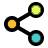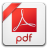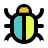Math Calculators, Lessons and Formulas

It is time to solve your math problem

mathportal.org

# Factor Trinomials Calculator

problem

Factor trinomial

$$\color{blue}{ 3x^2-5x+2 }$$

solution

The factored form is:

$$\color{blue}{ 3x^2-5x+2 = \left(x-1\right)\left(3x-2\right) }$$

explanation

Step 1: Identify constants $a$ , $b$ and $c$. ( $a$ is a number in front of the $x^2$ term $b$ is a number in front of the $x$ term and $c$ is a constant). In our case:

$$a = 3 , b = -5 ~ \text{ and } ~ c = 2$$

Step 2: Multiply the constant term by the leading coefficient.

$$a \cdot c = 6$$

Step 3: Find out two numbers that multiply to $a \cdot c = 6$ and add to $b = -5$.

Step 4: All pairs of numbers with a product of $6$ are:

 PRODUCT = 6 1     6 -1     -6 2     3 -2     -3

Step 5: Find out which factor pair sums up to $\color{blue}{ b = -5 }$

 PRODUCT = 6 and SUM = -5 1     6 -1     -6 2     3 -2     -3

Step 6: Replace middle term $-5 x$ with $-2x-3x$:

$$3x^{2}-5x+2 = 3x^{2}-2x-3x+2$$

Step 7: Apply factoring by grouping. Factor $x$ out of the first group and $-1$ out of the second group.

$$3x^{2}-2x-3x+2 = x\left(3x-2\right) -1\left(3x-2\right) = \left(x-1\right) \left(3x-2\right)$$

## Report an Error !

Script name : factoring-trinomials-calculator

Form values: 3 , 2 , 5 , 1 , 2 , g , Factor tinomial 3x^2-5x+2 = 0 , Factor 3x^2-5x+2

Comment (optional)

## Share Result

Or just copy and paste the link wherever you need it.Use this free step-by-step factoring calculator to factor a quadratic trinomial.
show help ↓↓ examples ↓↓ tutorial ↓↓
4x2 - 20x + 25
x2 - 8x + 15
2x2-11x+12
working...
EXAMPLES
example 2:ex 2:
Factor $16x^2 + 16x + 1$
example 2:ex 2:
Write trinomial $2 x^2 - 5x - 3$ in factored form.
example 2:ex 2:
Factor $6 x^2 +13x - 5$
Search our database of more than 200 calculators
TUTORIAL

## Polynomial Factoring Techniques

This calculator factors trinomials of the form $ax^2 + bx + c$ using the methods listed below.

1. Factoring perfect square trinomial

2. Factor if leading coefficient $a = 1$

3. Factor if leading coefficient $a \ne 1$

4. Special cases ( $b = 0$ ) or ( $a = 0$ )

### Method 1 : Factoring perfect square trinomial

Example 01: Factor $4a^2 - 12a + 9$

Step1: Verify that both the first and third terms are perfect squares.

$4a^2$ is perfect square because $4a^2 = \left(\color{blue}{2a}\right)^2$

$9$ is perfect square because $9 = \left(\color{red}{3}\right)^2$

Step2: Check if middle term is twice the product of $\color{blue}{2a}$ and $\color{red}{3}$

$$\text{middle term} = 12a = 2 \cdot \color{blue}{2a} \cdot \color{red}{3}$$

Step3: Put $\color{blue}{2a}$ and $\color{red}{3}$ inside parentheses. Because the middle term's coefficient is negative, we'll insert a minus sign inside parenthesis.

$$4a^2 - 12a + 9 = ( \color{blue}{2a} - \color{red}{3} )^2$$

### Method 2 : Leading coefficient $a = 1$

In this case, the trinomial has the following form $x^2 + bx + c$.

Example 02: Factor $x^2 + 7x + 10$

To factor this trinomial we need to find two integers ( $p$ and $q$ ) such that $p + q = b$ and $p \cdot q = c$.

In this example $p + q = 7$ and $p \cdot q = 10$

After some trials and errors we get $p = 2$ and $q = 5$

The factored form is

$$x^2 + 7x + 10 = ( x + p)(x + q) = (x + 2)(x + 5)$$

### Method 4 : Special Cases

Example 04: Factor $3x^2 + 5x$

This is special case where $c = 0$.

To solve this one we just need to factor $x$ out of $3x^2 + 5x$

$$3x^2 + 5x = x ( 3x + 5)$$

Example 05: Factor $25x^2 - 4$

This is special case where $b = 0$.

We'll need to use the difference of squares formula to factor this one.

$$25x^2 - 4 = (5x)^2 - 2^2 = (5x-2)(5x+2)$$

### Method 3 : Leading coefficient $a \ne 1$

In this case, the trinomial has the following form: $ax^2 + bx + c$.

Example 03: Factor $3x^2 - 5x + 2$

Step 1: Identify constants $a$ , $b$ and $c$

$$a = 3, b = -5 , c = 2$$

Step 2: Find out two numbers ( $p$ and $q$) that multiply to $a \cdot c = 6$ and add up to $b = -5$.

After some trials and errors we get $\color{blue}{p = -2}$ and $\color{red}{q =-3}$

Step 3:Replace middle term ( $-5x$ ) with $\color{blue}{-2}x \color{red}{-3}x$

$$3x^2 - 5x + 2 = 3x^2 - 2x - 3x + 2$$

Step 4:Factor out x from the first two terms and -1 from the last two terms.

\begin{aligned} 3x^2 - 5x + 2 &= 3x^2 - 2x - 3x + 2 = \\\\ &= x(3x-2) -1(3x-2) = \\\\ &=(x - 1)(3x-2) \end{aligned}
Search our database of more than 200 calculators